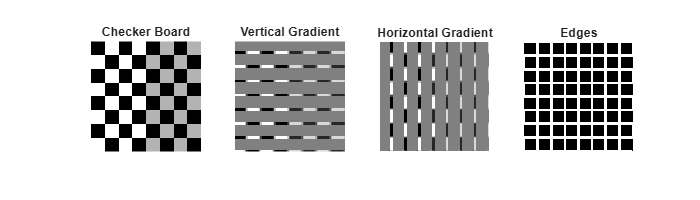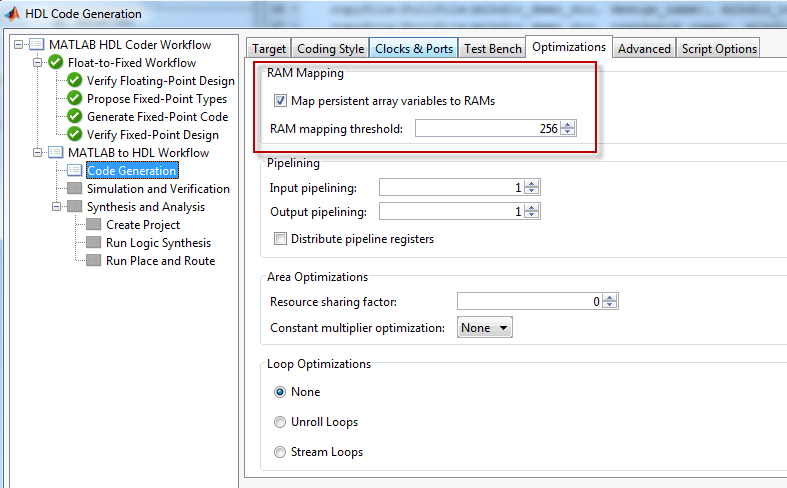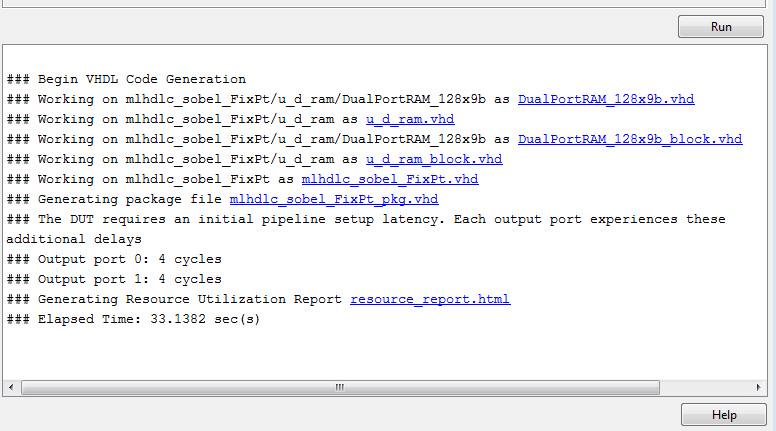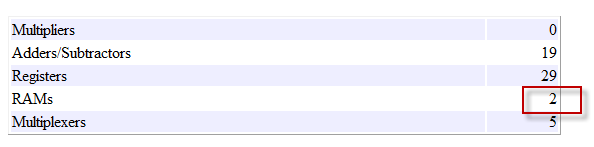# Map Matrices to Block RAMs to Reduce Area

This example shows how to use the RAM mapping optimization in HDL Coder™ to map persistent matrix variables to block RAMs in hardware.

### Introduction

One of the attractive features of writing MATLAB code is the ease of creating, accessing, modifying and manipulating matrices in MATLAB.

When processing such MATLAB code, HDL Coder maps these matrices to wires or registers in HDL. For example, local temporary matrix variables are mapped to wires, whereas persistent matrix variables are mapped to registers.

The latter tends to be an inefficient mapping when the matrix size is large, since the number of register resources available is limited. It also complicates synthesis, placement and routing.

Modern FPGAs feature block RAMs that are designed to have large matrices. HDL Coder takes advantage of this feature and automatically maps matrices to block RAMs to improve area efficiency. For certain designs, mapping these persistent matrices to RAMs is mandatory if the design is to be realized. State-of-the-art synthesis tools may not be able to synthesize designs when large matrices are mapped to registers, whereas the problem size is more manageable when the same matrices are mapped to RAMs.

### MATLAB Design

```design_name = 'mlhdlc_sobel'; testbench_name = 'mlhdlc_sobel_tb'; ```

### Create a New Folder and Copy Relevant Files

Execute the following lines of code to copy the example files into a temporary folder.

```mlhdlc_demo_dir = fullfile(matlabroot, 'toolbox', 'hdlcoder', 'hdlcoderdemos', 'matlabhdlcoderdemos'); mlhdlc_temp_dir = [tempdir 'mlhdlc_sobel']; % create a temporary folder and copy the MATLAB files cd(tempdir); [~, ~, ~] = rmdir(mlhdlc_temp_dir, 's'); mkdir(mlhdlc_temp_dir); cd(mlhdlc_temp_dir); % copy the design files to the temporary directory copyfile(fullfile(mlhdlc_demo_dir, [design_name,'.m*']), mlhdlc_temp_dir); copyfile(fullfile(mlhdlc_demo_dir, [testbench_name,'.m*']), mlhdlc_temp_dir); ```

### Simulate the Design

Simulate the design with the test bench prior to code generation to make sure there are no runtime errors.

```mlhdlc_sobel_tb ```### Create a New HDL Coder™ Project

Run the following command to create a new project.

```coder -hdlcoder -new mlhdlc_ram ```

Next, add the file 'mlhdlc_sobel.m' to the project as the MATLAB function, and 'mlhdlc_sobel_tb.m' as the MATLAB test bench.

Refer to Get Started with MATLAB to HDL Workflow for a more complete tutorial on creating and populating MATLAB HDL Coder projects.

### Turn On the RAM Mapping Optimization

The checkbox 'Map persistent array variables to RAMs' needs to be turned on to map persistent variables to block RAMs in the generated code.### Run Fixed-Point Conversion and HDL Code Generation

In the Workflow Advisor, right-click the 'Code Generation' step. Choose the option 'Run to selected task' to run all the steps from the beginning through HDL code generation.

### Examine the Generated Code

Examine the messages in the log window to see the RAM files generated along with the design.A warning message appears for each persistent matrix variable not mapped to RAM.

### Examine the Resource Report

Take a look at the generated resource report, which shows the number of RAMs inferred, by following the 'Resource Utilization report...' link in the generated code window.### Additional Notes on RAM Mapping

• Persistent matrix variable accesses must be in unconditional regions, i.e., outside any if-else, switch case, or for-loop code.

• MATLAB functions can have any number of RAM matrices.

• All matrix variables in MATLAB that are declared persistent and meet the threshold criteria get mapped to RAMs.

• A warning is shown when a persistent matrix does not get mapped to RAM.

• Read-dependent write data cycles are not allowed: you cannot compute the write data as a function of the data read from the matrix.

• Persistent matrices cannot be copied as a whole or accessed as a sub matrix: matrix access (read/write) is allowed only on single elements of the matrix.

• Mapping persistent matrices with non-zero initial values to RAMs is not supported.

### Clean up the Generated Files

Run the following commands to clean up the temporary project folder.

```mlhdlc_demo_dir = fullfile(matlabroot, 'toolbox', 'hdlcoder', 'hdlcoderdemos', 'matlabhdlcoderdemos'); mlhdlc_temp_dir = [tempdir 'mlhdlc_sobel']; clear mex; cd (mlhdlc_demo_dir); rmdir(mlhdlc_temp_dir, 's'); ```